## De Moivre's Theorem

The process of mathematical induction can be used to prove a very important theorem in mathematics known as De Moivre's theorem. If the complex number z = r(cos α + i sin α), then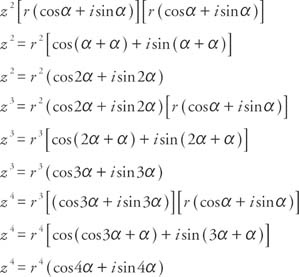The preceding pattern can be extended, using mathematical induction, to De Moivre's theorem.

If z = r(cos α + i sin α), and n is a natural number, thenExample 1: Write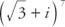in the form s + bi.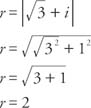Since cos α =and sin α = ½, α must be in the first quadrant and α = 30°. Therefore,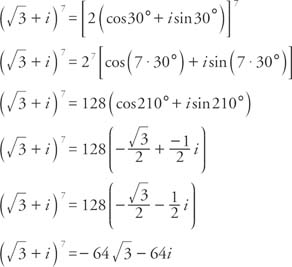Example 2: Write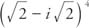in the form a + bi.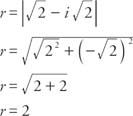Since cosand sin, α must be in the fourth quadrant and α = 315°. Therefore,Problems involving powers of complex numbers can be solved using binomial expansion, but applying De Moivre's theorem is usually more direct.

De Moivre's theorem can be extended to roots of complex numbers yielding the nth root theorem. Given a complex number z = r(cos α + i sinα), all of the nth roots of z are given by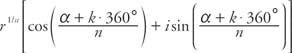where k = 0, 1, 2, …, (n − 1)

If k = 0, this formula reduces to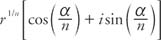This root is known as the principal nth root of z. If α = 0° and r = 1, then z = 1 and the nth roots of unity are given by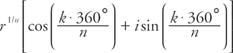where k = 0, 1, 2, …, ( n − 1)

Example 3: What are each of the five fifth‐roots of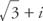expressed in trigonometric form?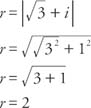Since cosand sin α = ½, α is in the first quadrant and α = 30°. Therefore, since the sine and cosine are periodic,and applying the nth root theorem, the five fifth‐roots of z are given by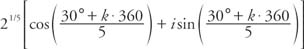where k = 0, 1, 2, 3, and 4

Thus the five fifth‐roots areObserve the even spacing of the five roots around the circle in Figure 1 .Figure 1
Drawing for Example 3.